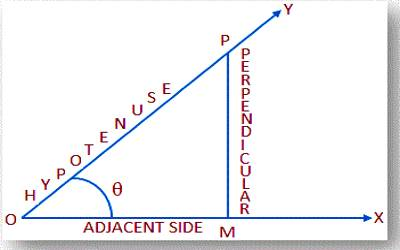“Trigon” is Greek for the triangle, and “metric” is Greek for measurement. The trigonometric ratios are special measurements of a right triangle (a triangle with one angle measuring 90°). Remember that the two sides of a right triangle which form the right angle are called the legs, and the third side (opposite the right angle) is called the hypotenuse.

• The sine of the angle is the ratio of the length of the side opposite the angle divided by the length of the hypotenuse. It is the trigonometric function that is equal to the ratio of the side opposite a given angle (in a right-angled triangle) to the hypotenuse.
• The cosine of the angle is the ratio of the length of the side adjacent to the angle divided by the length of the hypotenuse. It is the trigonometric function that is equal to the ratio of the side adjacent to an acute angle (in a right-angled triangle) to the hypotenuse.
• The tangent of the angle is the ratio of the length of the side opposite the angle divided by the length of the side adjacent to the angle.

Limit of Trigonometric Ratios

In the limit of trigonometric ratios, we will learn how to find the limits to the values of sin θ, csc θ, cos θ, sec θ, tan θ and cot θ.

According to the definitions of the trigonometrical ratios of a positive acute angle are always positive.

Note: Remember that the trigonometrical ratios may be positive as well as negative.We get from the definitions of trigonometrical ratios that,

Sin θ = PM/OP and Cos θ = OM/OP …….. (A)

From the above picture, OP is the hypotenuse of the triangle POM; hence, PM ≮ OP and OM ≮ OP.

Therefore, from (A) we get the values of sin θ and cos θ cannot be greater than 1.

Again, csc θ = OP/PM and sec θ = OP/OM

Therefore, it is clearly seen that the values of csc θ and sec θ can never be less than 1.

Finally, tan θ = PM/OM and cot θ = OM/PM.

In this case, the values of PM may be greater or less or equal to the values of OM. Thus, the values of tan θ or cot θ may have any non-negative value.

Therefore, the limit of trigonometric ratios of a positive acute angle θ is always non-negative:

(i) The values of sin θ and cos θ cannot be greater than 1;

(ii) The values of csc θ and sec θ cannot be less than 1; and

(iii) The values of tan θ and cot θ can have any value.

Information Source: